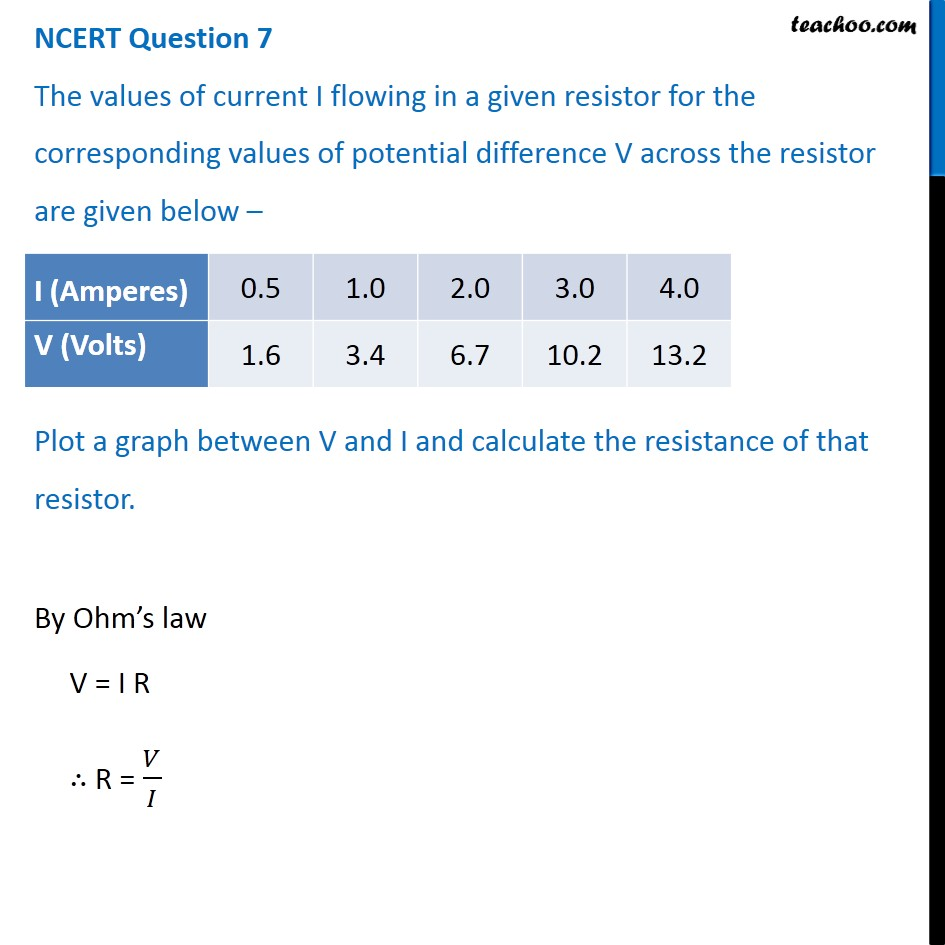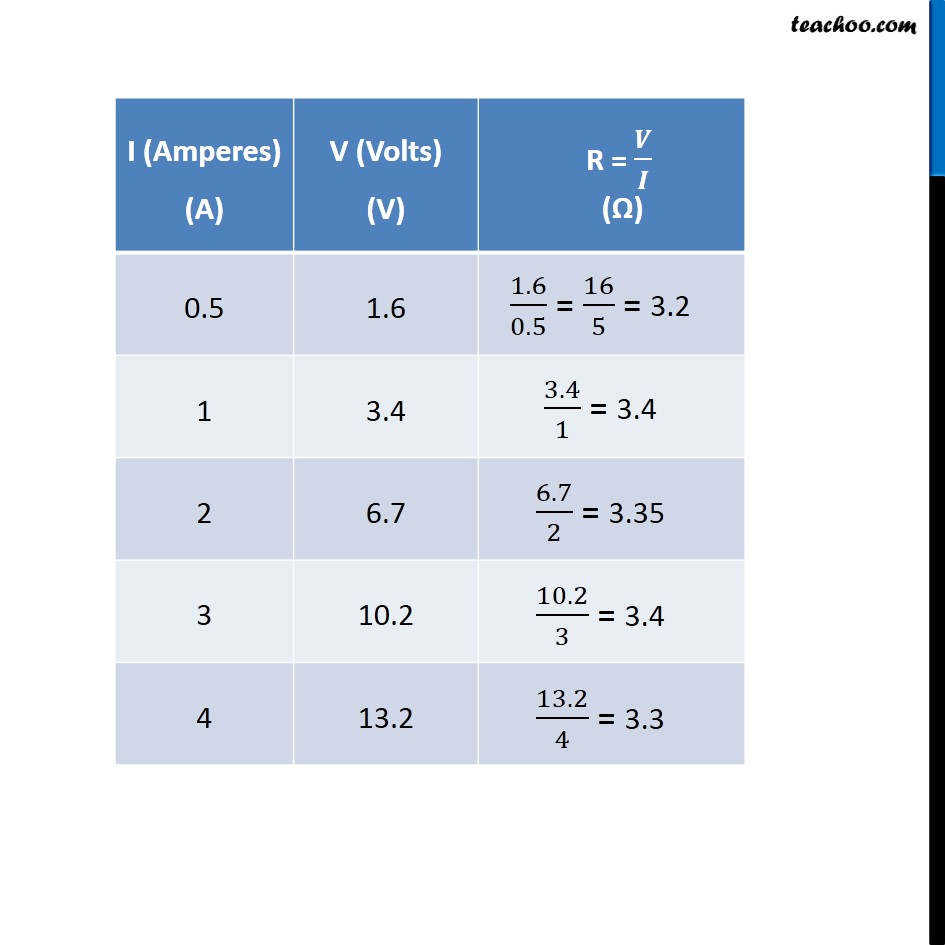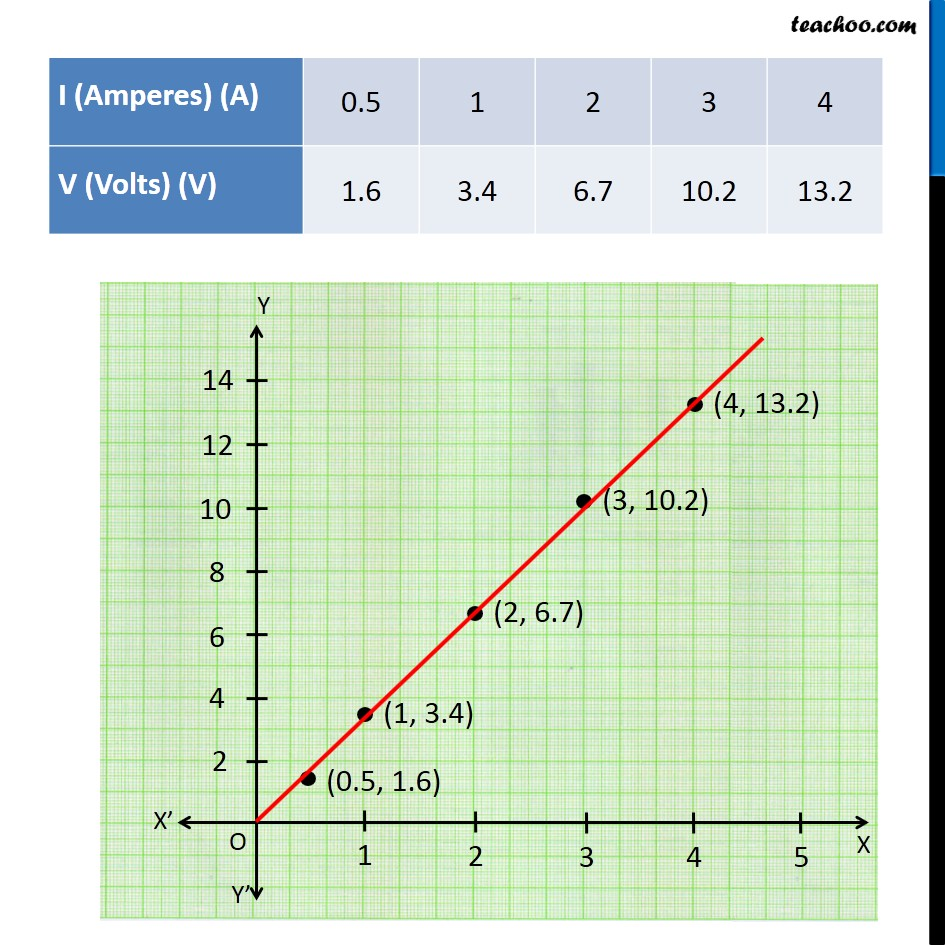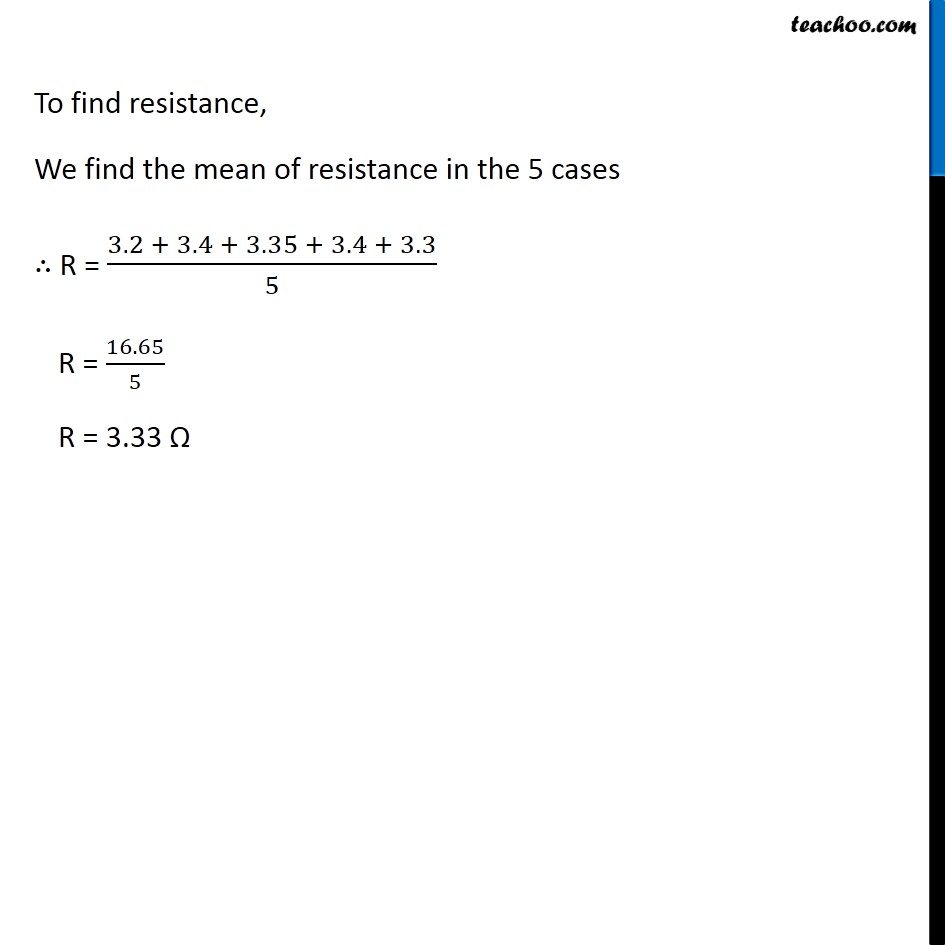NCERT Questions

Class 10
Chapter 12 Class 10 - Electricity

## Plot a graph between V and I and calculate the resistance of that resistor.Get live Maths 1-on-1 Classs - Class 6 to 12

### Transcript

NCERT Question 7 The values of current I flowing in a given resistor for the corresponding values of potential difference V across the resistor are given below – Plot a graph between V and I and calculate the resistance of that resistor. By Ohm’s law V = I R ∴ R = 𝑉/𝐼 To find resistance, We find the mean of resistance in the 5 cases ∴ R = (3.2 + 3.4 + 3.35 + 3.4 + 3.3)/5 R = 16.65/5 R = 3.33 Ω